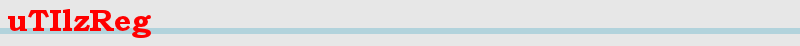Index uTIlzreg - uTIlzReg_LDDMM_Beg - uTIlzReg_GeoShoot - uTIlzReg_Demons - uTIlzReg_Tools

uTIlzReg is for research purposes only and has not been approved for clinical use.

### Presentation:

uTIlzReg_LDDMM_Beg is a program to perform LDDMM registration between 2D/3D images. Its main goal is to compare images with initial momenta. It can perform multi-kernel image registration (Risser TMI 2011). The computations of uTIlzReg_LDDMM_Beg are done in the source (= template) image coordinate system. Remark that our implementation is not the original JHU one, so it may lead to slighly different results.

### References:

LDDMM Registration algorithm: Beg F., Miller M., Trouvé A., Younes L., Computing Large Deformation Metric Mappings via Geodesic Flows of Diffeomorphisms. International Journal of Computer Vision, 61(2); 2005

Multi-kernel LDDMM registration: Risser L., Vialard F.X., Wolz R., Murgasova M., Holm D., Rueckert D., ADNI: Simultaneous Multiscale Registration using Large Deformation Diffeomorphic Metric Mapping. IEEE Transactions on Medical Imaging 30(10); 2011

### Calling the function:

./uTIlzReg_LDDMM_Beg [Source] [Target] <options>

where [Source] and [Target] are the source (moving) and target (fixed) images, and <options> are obviously the options.

### Options:

#### Primary options:

<-iterations n> Number of iterations (default=10)

<-subdivisions n> Number of subdivisons (default=10)

<-MaxVeloUpdt n> Maximum velocity update at each iteration (default=0.4 voxels)

#### Inputs and Outputs:

<-PrefixInputs n> Prefix of the files containing an initial velocity field (default="Null")

<-PrefixOutputs n> Prefix of the files containing the outputs (default="Outputs")

<-AddChannel W S T> Add a channel -> W=weight (wgt of ref channel is 1) S=Source T=Target

<-Mask n> Definition of a mask (default="Null")

<-affineT n> Affine transfo from Trg to Src in the world domain. The 4*3 parameters are: r_xx r_xy r_xz t_x r_yx ... t_z

#### Kernels (Default: -Gauss 1):

<-Gauss S> Gaussian kernel (S = std. dev. in mm)

<-M_Gauss n> Sum of Gaussian kernels (max 7) -- n = k W1 S1 ... Wk Sk (k=[#kernels], W.=weight)

<-M_Gauss_easy n> Sum of Gaussian kernels (max 7) with apparent weights = 1 -- n = k S1 ... Sk (k=[#kernels])

<-M_Gauss_easier n> Sum of 7 linearly sampled Gaussian kernels with apparent weights = 1 -- n = Smax Smin

#### Secondary options:

<-TranslatEstim> Allows translations of the target

<-symmetric> Symmetric registration

<-epsilon n> Threshold on the normalized max update of the velicty field (default=0.2)

<-GreyLevAlign n> Grey level linear alignment of each channel (Inputs: Padding Src - Padding Trg)

<-margins n> Margin of the image where the calculations are reduced (default=0 voxels)

<-WghtVeloField n> Weight of the velocity field in the energy (default=0.001)

<-RefMaxGrad n> Value to manage the convergence. Automatically configured if <0 (default=-1.)

#### Special Outputs:

<-FinalDefVec> Displacement field in mm from [Source] to [Target] (by default for the whole deformation)

<-FinalDefInvVec> Displacement field in mm from [Target] to [Source]

<-SplitKernels> Split the contribution of each kernel

<-AOD> Amplitude of the deformations from each voxel of the source image

<-DetJacobian> Determinant of the Jacobian at each voxel

<-InitMomentum> Estimated initial momentum

<-ShowSSD> Show the Sum of the Squared Differences at t=1 ieration after iteration

Main menu